# MCQs on Power Systems

##### Page 34 of 67. Go to page 1 2 3 4 5 6 7 8 9 10 11 12 13 14 15 16 17 18 19 20 21 22 23 24 25 26 27 28 29 30 31 32 33 34 35 36 37 38 39 40 41 42 43 44 45 46 47 48 49 50 51 52 53 54 55 56 57 58 59 60 61 62 63 64 65 66 67
01․ The positive (Z1), negative (Z2) and zero sequence (Z0) impedances of a transmission line follows the relation
Z1 = Z2 = Z0
(Z1 = Z2) < Z0
(Z1 = Z2) > Z0
any of the above

Zero sequence impedance Z0 = Zs + 2 Zm Positive sequence impedance Z1 = Negative sequence impedance Z2 = Zs - Zm Therefore, (Z1 = Z2) < Z0 In general zero sequence current is three or four times of the positive or negative sequence impedance of the line.

02․ Peterson coil is used in case of transmission line for
reduce ferranti effect
compensation of arcing grounds
improve power factor
increase the voltage level

In case of ground faults LG and LLG, arcing grounds are produced, this will cause charging current of the capacitor increase and hence voltage of the healthy phases are raised to dangerous limits, at which insulation failure of healthy phases occurs. To compensate this effect an inductive coil called 'Peterson coil' is connected between ground and the neutral. The value of inductance used for compensation of arcing grounds = 1/3ω²C

03․ Which of the following represents the transmission line positive sequence reactance
Xs - Xm
Xm - Xs
Xs +2 Xm
2Xs + Xm

For a transmission line, Zero sequence impedance Z0 = Zs + 2 Zm Positive sequence impedance Z1 = Negative sequence impedance Z2 = Zs - Zm

04․ High capacity generators and motors are of
delta connected network
star connected network
star connected with grounding network
any of the above

In case of delta network, there is no path for zero sequence currents because there is no ground return and hence considered zero sequence currents are circulating within the winding. High capacity generators and motors of star connected with grounding is practically used for faster identification of faults and to isolate the faulty section.

05․ Which of the following statements is/are true? In case of parallel transmission lines
stability increases
fault current reduces
fault current increases
both 1 and 3

If the fault is very nearer to the substation or generator, the reactance of the network is small. So that fault current is larger. Fault current If ∝ 1/X (inversely proportional to reactance) Where X = Reactance of the network Therefore, compare to the single transmission line, parallel line or double line has low reactance. So that the fault current is more in the case of parallel lines. As load angle is directly proportional to transmission line reactance value, load angle is less for parallel lines, this will increases the stability of the transmission line.

06․ The positive, negative and zero sequence per unit impedance of two generators connected in parallel are X1 = 0.12 pu, X2 = 0.096 pu and X0 = 0.036 pu. For LG fault at generator terminals (with 1 pu voltage) the positive sequence current will be
10.936 pu
7.936 pu
8.936 pu
9.936 pu

For LG fault at generator terminals, the positive sequence current IR1 = V/(X1+X2+X0)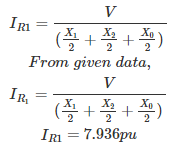Since, two generators with same X1, X2 and X0 are connected in parallel,

07․ The VSWR of transmission line is
Proportional to load and characteristic impedance.
Directly proportional to voltage minimum and inversely proportional to voltage maximum.
Directly proportional to load impedance and inversely to characteristic impedance.
Directly proportional to characteristic impedance and inversely to load impedance.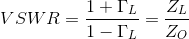08․ The SWR on a lossless transmission line of the characteristic impedance 100 ohm is 3. The line is the terminated by
A resistance of 100/3 ohm.
A resistance of 100 ohm.
A resistance of j300 ohm.
A resistance of j100/3 ohm.

For a transmission line, reflection co-efficient,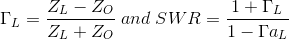Here ZL = 3 00 ΓL = 200/400 = ½ and SWR = 3.

09․ For a line characteristic impedance zo terminated is load zo/3, the reflection coefficient is
1/3.
– ½.
– 1/3.
2/3

ZL = Z0/3,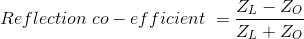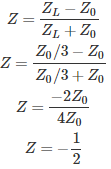10․ If R = 84 ohm/Km, G = 10-6 mho/Km, H = 0.01 H/Km, C = 0.061 μ F/Km and frequency = 1000 Hz, then what is the value of propagation constant of the transmission line
0.01 + j 0.02 per Km.
0.09 + j 0.018 per Km.
0.018 per Km.
None of them.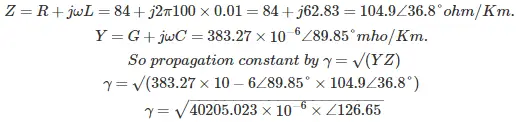<<<3233343536>>>